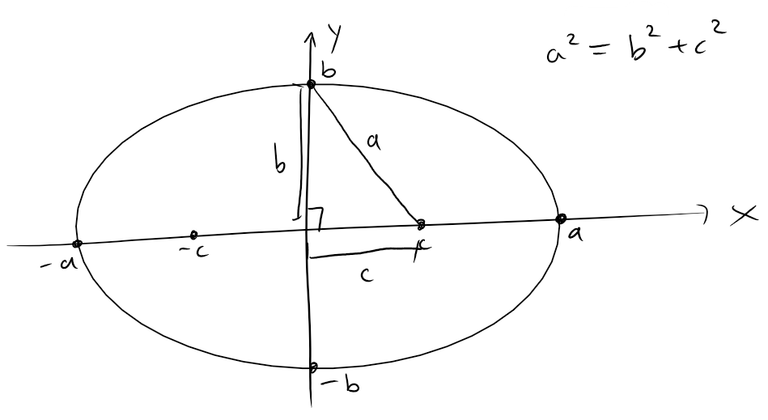# Ellipses: Definition and Proof of Equation

in #mathematics11 months ago (edited)

In this video I go over the definition of an ellipse as well as go over a pretty extensive analysis of the proof for its equation. Lots of real world applications involve ellipses such as the orbits of planets and stars so it is important to fully understand the equation and properties of ellipses.

Watch Video On:

# View Video Notes Below!

View These Notes as an Article: https://steemit.com/@mes
Subscribe via Email: http://mes.fm/subscribe
Donate! :) https://mes.fm/donate

Reuse of My Videos:

• Feel free to make use of / re-upload / monetize my videos as long as you provide a link to the original video.

Fight Back Against Censorship:

• Bookmark sites/channels/accounts and check periodically
• Remember to always archive website pages in case they get deleted/changed.

Join my private Discord Chat Room: https://mes.fm/chatroom

Check out my Reddit and Voat Math Forums:

Buy "Where Did The Towers Go?" by Dr. Judy Wood: https://mes.fm/judywoodbook
Follow My #FreeEnergy Video Series: https://mes.fm/freeenergy-playlist
Watch my #AntiGravity Video Series: https://steemit.com/antigravity/@mes/series

• See Part 6 for my Self Appointed PhD and #MESDuality Breakthrough Concept!

Follow My #MESExperiments Video Series: https://steemit.com/mesexperiments/@mes/list

NOTE #1: If you don't have time to watch this whole video:

NOTE #2: If video volume is too low at any part of the video:

# Ellipses: Definition and Proof of Equation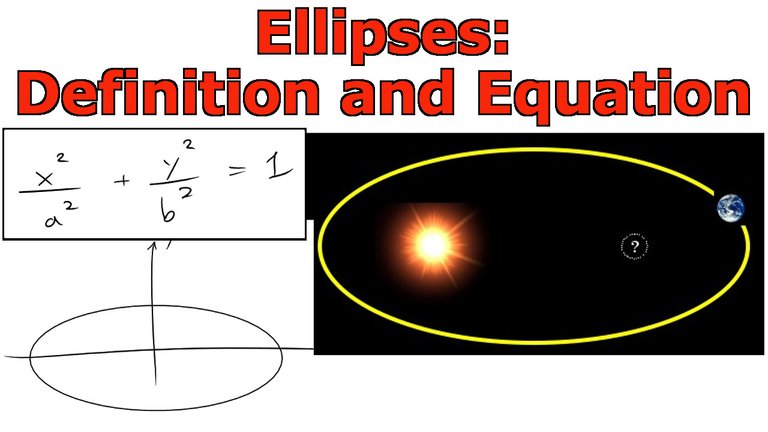Equation of an ellipse: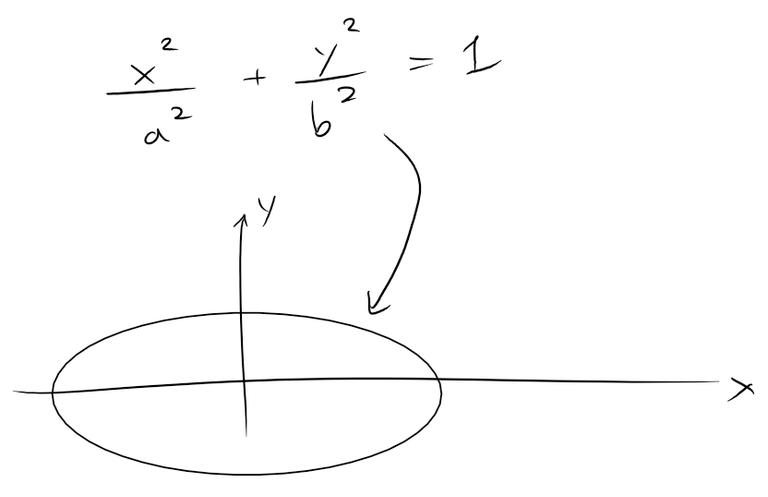Definition: An ellipse is the set of points in a plane the sum of whose distances from two fixed points F1 and F2 is a constant.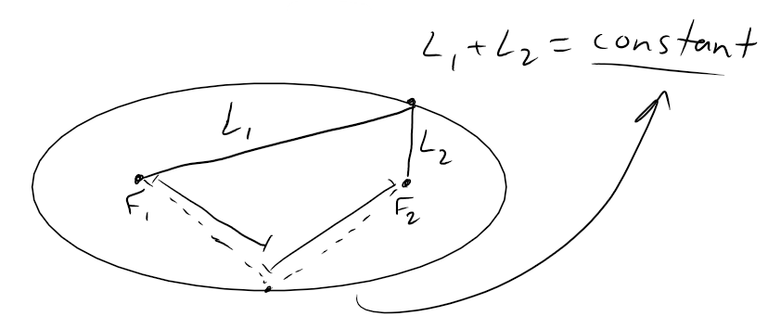These two fixed points are called the foci (plural of focus).

One of Johannes Kepler's laws is that the orbits of the planets in the solar system are ellipses with the Sun at one focus.

Kepler was a 17th century German astronomer and mathematician and was a key figure in the 17th century scientific revolution and whose works laid one of the foundations for Isaac Newton's theory of universal gravitation.

In order to obtain the equation of an ellipse, we place the foci on the x-axis (-c, 0) and (c, 0) so that the origin is halfway between the foci: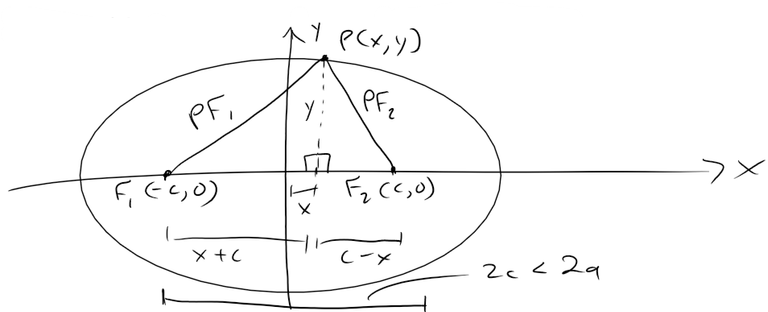Let the sum of the distances from a point on the ellipse to the foci be 2a > 0. Then P(x, y) is a point on the ellipse when: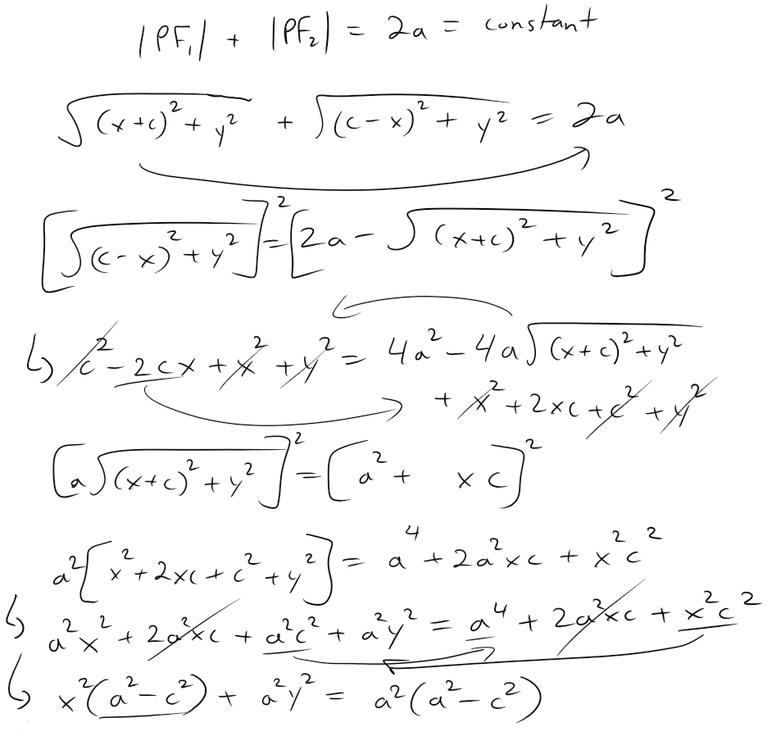From the above figure, notice that: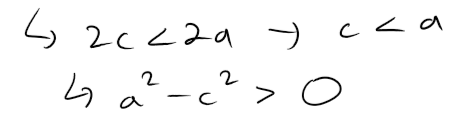For convenience, let b2 = a2 - c2. Then the equation of the ellipse becomes: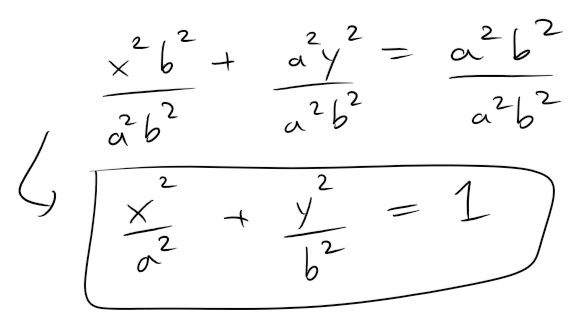Since b2 = a2 - c2 then: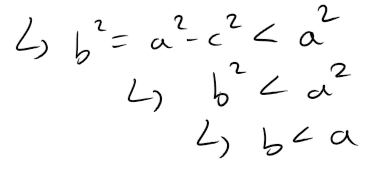The x-intercepts are found by setting: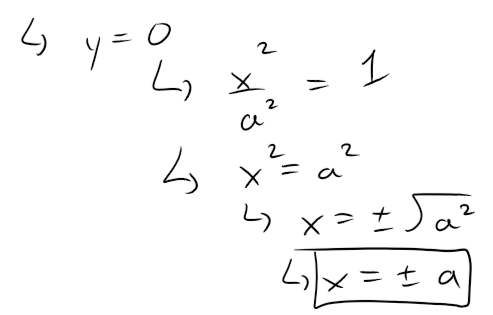The corresponding points (a, 0) and (-a, 0) are called the vertices of the ellipse and the line segment joining the vertices is called the major axis.

To find the y-intercepts, we set: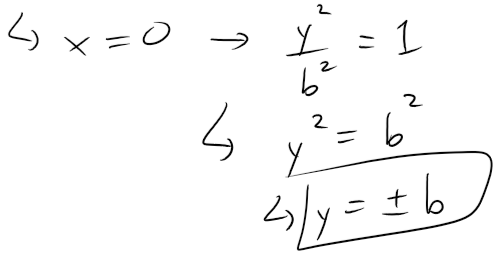The equation of an ellipse is unchanged if x is replaced by -x or y is replaced by -y, so the ellipse is symmetric about both axes.

Notice that if the foci coincide, then c = 0, so a = b and the ellipse becomes a circle with radius r = a = b: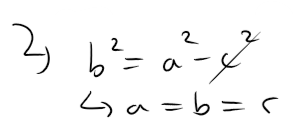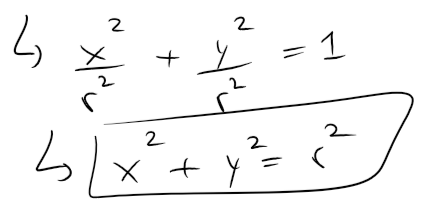In summary:

The equation of an ellipse is: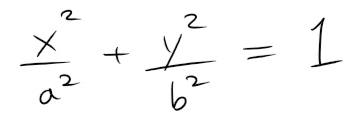And has foci (+/- c, 0) where c2 = a2 - b2, and vertices (+/- a, 0).